Next: Quantum Interference of Light Up: Wave-Particle Duality Previous: Quantum Theory of Light

# Classical Interference of Light Waves

Let us now consider the classical interference of light waves. Figure 5 shows a standard double-slit interference experiment in which monochromatic plane light waves are normally incident on two narrow parallel slits which are a distance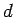apart. The light from the two slits is projected onto a screen a distance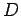behind them, where.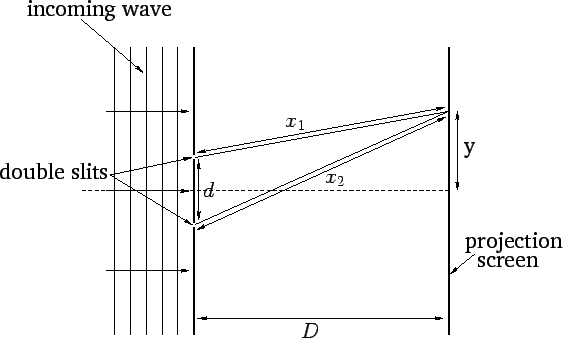Consider some point on the screen which is located a distancefrom the centre-line, as shown in the figure. Light from the first slit travels a distanceto get to this point, whereas light from the second slit travels a slightly different distance. It is easily demonstrated that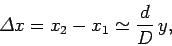(63)

provided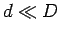. It follows from Eq. (46), and the well-known fact that light waves are superposible, that the wavefunction at the point in question can be written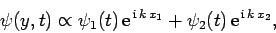(64)

where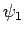and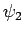are the wavefunctions at the first and second slits, respectively. However,(65)

since the two slits are assumed to be illuminated by in-phase light waves of equal amplitude. (Note that we are ignoring the difference in amplitude of the waves from the two slits at the screen, due to the slight difference betweenand, compared to the difference in their phases. This is reasonable provided.) Now, the intensity (i.e., the energy flux) of the light at some point on the projection screen is approximately equal to the energy density of the light at this point times the velocity of light (provided that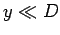). Hence, it follows from Eq. (56) that the light intensity on the screen a distancefrom the center-line is(66)

Using Eqs. (63)-(66), we obtain(67)

Figure 6 shows the characteristic interference pattern corresponding to the above expression. This pattern consists of equally spaced light and dark bands of characteristic width(68)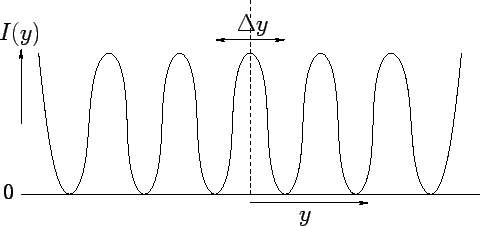Next: Quantum Interference of Light Up: Wave-Particle Duality Previous: Quantum Theory of Light
Richard Fitzpatrick 2010-07-20